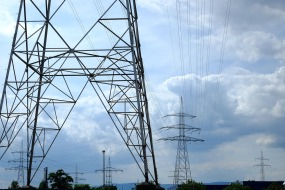## Unit 1: Motion (Kinematics)

### Overview

In this unit, you will learn about objects in motion. You will apply terminology such as distance, position, displacement, speed, velocity, and acceleration in the correct context. You will learn how to create and interpret distance-time, position-time, and velocity-time graphs. You will investigate objects in motion and solve problems using kinematics equations.## Unit 2: Forces and Newton's Laws of Motion

### Overview

In this unit, you will learn about different types of forces and how they affect the motion of an object. You will learn about Newton’s Laws and apply them to analyze the motion of an object. You will create and interpret free body diagrams and solve problems involving Newton’s Laws, gravitation, and friction as needed to describe the motion of an object.## Unit 3: Work, Energy, and Power

### Overview

In this unit, you will learn about concepts related to work, energy, and power. You will learn about the principle of conservation of energy and analyze objects in terms of their gravitational potential and kinetic energy in order to solve problems. You learn about energy transformations and identify situations in which energy is transformed into less useful forms, and calculate the efficiency of a system.## Unit 4: Simple Machines

### Overview

In this unit, you will learn about simple machines such as levers, pulleys, and inclined planes. You will apply terminology such as effort arm, load arm, ideal mechanical advantage, and actual mechanical advantage appropriately. You will solve problems involving simple machines and analyze their efficiency.## Unit 5: Hydraulics and Pneumatics

### Overview

In this unit, you will learn about factors affecting static and dynamic fluid systems. You will solve problems involving pressure and density and apply Pascal’s Principle to make predictions about pressure, forces, areas, and distances in hydraulic systems. You will analyze hydraulic systems with respect to the speed with which they can operate. You will be able to compare hydraulic and pneumatic systems and select the best type of system for a particular application.## Unit 6: Electricity and Magnetism

### Overview

In this unit, you will learn about current, potential difference, and resistance in electrical circuits. You will solve problems using Ohm’s Law, Kirchhoff’s Current Law, and Kirchhoff’s Voltage Law. You will learn about different magnetic properties and phenomena and be able to describe the magnetic field around a permanent magnet, straight current-carrying conductor, and solenoid. You will apply the motor principle to predict the direction of the electromagnetic force on a straight current-carrying conductor in an external magnetic field.## Unit 7: Course Culminating Activity

### Overview

In this unit, you will consolidate the concepts and skills you have learned throughout this course. You will complete the course culminating activity, through which you will analyze the impacts of energy transformation technologies on society and the environment.

### Unit 7 Activities

Activity 1: Course Culminating Activity